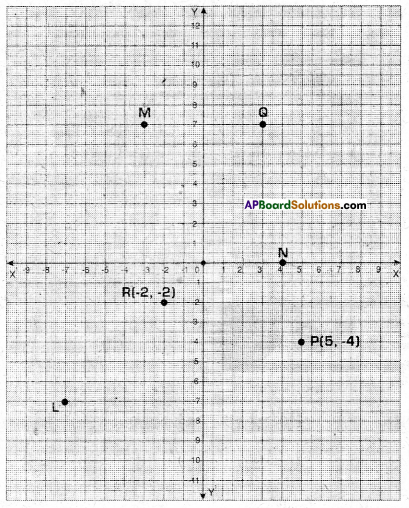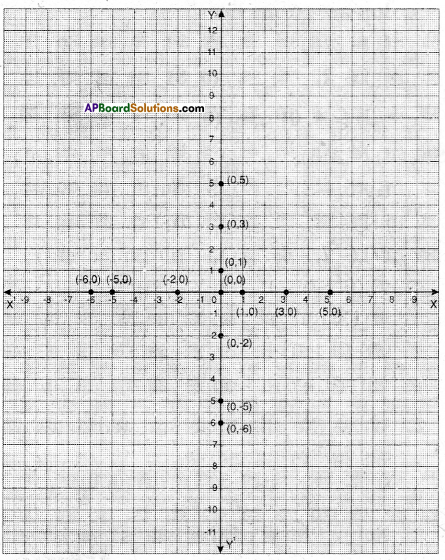# AP Board 9th Class Maths Solutions Chapter 5 Co-Ordinate Geometry Ex 5.2

AP State Syllabus AP Board 9th Class Maths Solutions Chapter 5 Co-Ordinate Geometry Ex 5.2 Textbook Questions and Answers.

## AP State Syllabus 9th Class Maths Solutions 5th Lesson Co-Ordinate Geometry Exercise 5.2

Question 1.
Write the quadrant in which the following points lie.
i) (- 2, 3)
ii) (5, – 3)
iii) (4, 2)
iv) (- 7, – 6)
v) (0, 8)
vi) (3, 0)
vii) (-4,0)
viii) (0, – 6)
Solution:
i) (- 2, 3) – Q2 (second quadrant)
ii) (5, – 3) – Q4 (fourth quadrant)
iii) (4, 2) – Q1 (Iirst quadrant)
iv) (- 7, – 6) – Q3 (third quadrant)
v) (0, 8) – on Y-axis
vi) (3, 0) – on X-axis
vii) (-4,0) – on X’ – axis
viii) (0, – 6) – on Y’: axisQuestion 2.
Write the abscissae and ordinates of the following points.
i)(4,-8)
ii)(-5,3)
iii)(0,0)
iv)(5, 0)
v)(0, -8)
Note: Plural of abscissa is abscissae.
Solution:

 Point abscissa ordinate i) (4, – 8) 4 -8 ii)  (-5, 3) -5 3 iii) (0,0) 0 0 iv) (5,0) 5 0 v) (0,-8) . 0 -8Question 3.
Which of the following points lie on the axes ? Also name the axis.
i) (-5,-8)
ii) (0, 13)
iii) (4, – 2)
iv) (- 2,0)
v) (0, – 8)
vi) (7,0)
vii) (0,0)
Solution:
The points (ii) (0,13) ; (v) (0,- 8) lie on Y – axis.
The points (iv) (- 2, 0), (vi) (7, 0) lie on X – axis.
The point (vii) (0, 0) lie on both X – axis and Y – axis.
The points (i) (- 5, – 8); (iii) (4, – 2) do not lie on any axis.

Question 4.
Write the following based on the graph.
i) The ordinate of L
ii) The ordinate of Q
iii) The point denoted by (- 2,-2)
iv) The point denoted by (5, – 4)
v) The abscissa of N
vi) The abscissa of MSolution:
i) – 7
ii) 7
iii) The point ’R’
iv) The point P
v) 4
vi) – 3Question 5.
State true or false and write correct statement.
i) In the Cartesian plane the horizon-tal line is called Y – axis, if) in the Cartesian plane, the vertical line is called Y – axis.
iii) The point which lies on both the axes is called origin.
iv) The point (2, – 3) lies in the third quadrant.
v) (-5, -8) lies in the fourth quadrant.
vi) The point (- x, – y) lies in the first quadrant where x < 0; y < 0.
Solution:
i) False
Correct statement: In the Cartesian plane the horizontal line is called X – axis.
ii) True
iii) True
iv) False
Correct statement: The point (2, -3) lies in the fourth quadrant.
v) False
Correct statement: (- 5, – 8) lies in the third quadrant.
vi) TrueQuestion 6.
Plot the following ordered pairs on a graph sheet. What do you observe ?
i) (1, 0), (3, 0), (- 2, 0), (- 5, 0), (0, 0), (5, 0), (- 6, 0)
ii) (0, 1), (0, 3), (0, – 2), (0, – 5), (0, 0), (0,5), (0,-6)
Solution:
i) All points lie on X – axis,
ii) All points lie on Y – axis.